Courses

# Test: Alkanes And Cycloalkanes

## 22 Questions MCQ Test Chemistry Class 11 | Test: Alkanes And Cycloalkanes

Description
This mock test of Test: Alkanes And Cycloalkanes for JEE helps you for every JEE entrance exam. This contains 22 Multiple Choice Questions for JEE Test: Alkanes And Cycloalkanes (mcq) to study with solutions a complete question bank. The solved questions answers in this Test: Alkanes And Cycloalkanes quiz give you a good mix of easy questions and tough questions. JEE students definitely take this Test: Alkanes And Cycloalkanes exercise for a better result in the exam. You can find other Test: Alkanes And Cycloalkanes extra questions, long questions & short questions for JEE on EduRev as well by searching above.
QUESTION: 1

### Direction (Q. Nos. 1 - 11) This section contains 11 multiple choice questions. Each question has four choices (a), (b), (c) and (d), out of which ONLY ONE option is correct. Q. When light is shined on a mixture of chlorine and ethane, chloroethane is formed besides dichloroethane, trichloroethane and several other products. What reaction condition can optimise the yield of chloroethane?

Solution:

When chlorine and Ethane are taken with chlorine in excess only then we have more than one product like chloroethane, dichloroethane, trichloroethane etc. To avoid this we should take Ethane in excess because when we will take it then in excess then we will have only single time chlorination and we will get monochloroethane.

QUESTION: 2

### Which of the following is not a possible termination step in the free radical chlorination of methane?

Solution:

Termination is the last step. So there shouldn't be any free radical atom remaining. In first option there is Cl• remaining it can't be termination step.The steps in free radical halogenation are as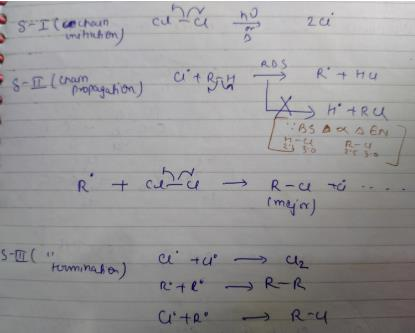QUESTION: 3

### The major monobromination product which results when ethyl cyclohexane is subjected to free radical bromination, is

Solution:

It's a tertiary bromide . Br2 converts into Br+ Be because of free radical abstracts Br form new Br2 to continue the chain

QUESTION: 4

What is relative reactivity of secondary versus primary hydrogens in free radical bromination of n-butane if the ratio of 1-bromo to 2-bromobutane formed is 7 : 39?

Solution:

Explanation : The 2o hydrogens are 20 times more reactive than the 1o ones.

QUESTION: 5

What is the major monobromination product in the following reaction?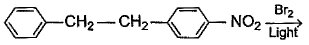Solution:

Bromination in the presence of light is done by free radical mechanism. The radical on the carbon chain is formed where the radical is most stable. We can’t put radical on the ring as the aromaticity of the ring will be lost. So, it should be put either on the CH3. However, the CH3 which is attached to NO2 ring will not bear stable radical as NO will decrease the stability of radical.(NO2 is an electron withdrawing group). So the only position is the other CH3 in option d. There also we have stability due to resonance.

QUESTION: 6

Which is the best description of Hammond postulate?

Solution:

Hammond's postulate states that the transition state of a reaction resembles either the reactants or the products, to whichever it is closer in energy. In an exothermic reaction, the transition state is closer to the reactants than to the products in energy

QUESTION: 7

What is the major bromination product in the following reaction?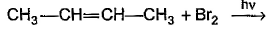Solution:

The correct answer is option c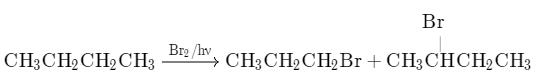Thus, a racemic mixture is obtained. A racemic mixture is one that has an equal amount of left and right handed enantiomers of a chiral molecule.

QUESTION: 8

During free radical bromination of isobutane, an alkene is produced as by product via disproportionation of the intermediate alkyl free radical. What is this alkene?

Solution: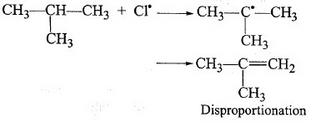As shown in the above mechanism 1-butene and 2-butene cannot be formed by this free radical. release of 1 hydrogen radical gives 2-methyl propene as disproportionated product.

Hence, the correct option is C.

QUESTION: 9

How many dichlorocyclohexane would be produced upon free radical chlorination of chlorocyclohexane?

Solution:

Product 1-Does not have any chiral carbon so only one product
Product 2-Two chiral centres so RR, RS and SR possible
Product 3-Two chiral centres so RR, RS and SR possible
Product 4-Two chiral centres but plane of symmetry exists, so only two isomers.

QUESTION: 10

Which of the following statem ents regarding free radical halogenation of alkane is not true?

Solution:

Hydrogen abstraction by halogen radical in the propagation step is exothermic in chlorination but endothermic in bromination. Hence, the option (A) is an incorrect statement.

QUESTION: 11

Arrange the following in increasing order of boiling points.

I. 3 -methyl pentane
II. 3-chloropentane
III. 3-bromopentane
IV. 3,3-dichloropentane

Solution:

For boiling point, we have to consider both branching and Molecular mass. In 4  bromopentane molecular mass is nearly the same as compared to 3 chloro pentane but we have 3,3-dichloropentane extended into 2 directions so the boiling point of 3,3-dichloropentane will be more and the other order will be followed by option C.

*Multiple options can be correct
QUESTION: 12

Direction (Q. Nos. 12 -15) This section contains 4 multiple choice questions. Each question has four choices (a), (b), (c) and (d), out of which ONE or MORE THAN ONE are correct.

Q. In the following free radical bromination reaction, the important product(s) is/are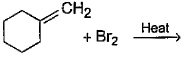Solution:

This is the case of allylic substitution. The radical will delocalise itself to two locations. And so, we will get two different positions for radical substitution.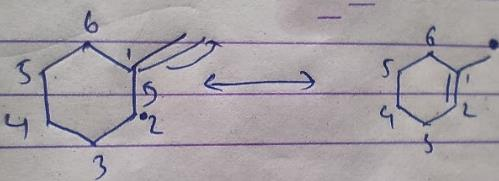There is also a possibility at C6, but it is not in our option.

*Multiple options can be correct
QUESTION: 13

What is/are true regarding free radical iodination of an alkane?

Solution:

The statements (B) and (C) are true regarding free radical iodination of the alkane.
(B) Direct iodination of alkane with iodine in presence of light is impractical.
During this reaction, Hl, a strong reducing agent is obtained as a byproduct, which catalyzes the reverse reaction thereby preventing direct iodination of alkane.
(C) Iodination of alkane can be achieved successfully using an oxidizing agent catalyst.
Presence of an oxidizing agent oxidizes unwanted byproduct Hl and enables iodination of alkane to proceed.

*Multiple options can be correct
QUESTION: 14

Consider the following bromination reaction.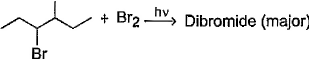If a pure enantiomer of reactant is taken in the above reaction, the correct statement concerning product dibromide is/are

Solution:
*Multiple options can be correct
QUESTION: 15

Which of the following reactions can bring about chlorination of cyclohexane?

Solution:

For SO2Cl2: The reactivity patterns of SO2Cl2 and SOCl2 are quite different. SOCl2 is a good electrophile, and can be thought of as a source of Cl− ions. These ions can go on to react in their typical nucleophilic fashion. SO2Cl2 however is often a Cl2 source, as it readily decomposes giving off sulfur dioxide. Usually, much easier/safer to use this than measuring out (and getting into solution) chlorine gas. The chlorination of simple alkanes by Cl2 gas (or something that makes it in solution) happens by a radical mechanism i.e. Cl⋅ not Cl
For Cl2 and heat/light: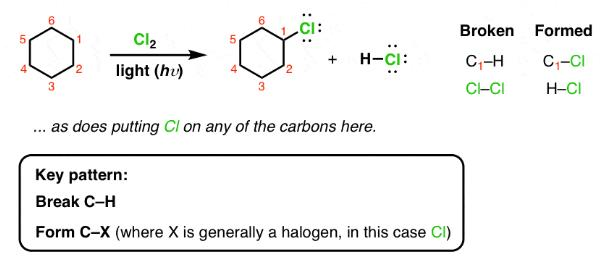For Cl with AlCl3: It is used for chlorination of compounds like benzene
For HCl: It is used for halogenations of a double bond.

QUESTION: 16

Direction (Q. Nos. 16 - 18) This section contains a paragraph, describing theory, experiments, data, etc.
Three questions related to the paragraph have been given. Each question has only one correct answer among the four given options (a), (b), (cj and (d).

Passage

A hydrocarbon with molecular formula C10H18, upon catalytic hydrogenation gives C10H20 (X). X on free radical chlorination gives two monochloro derivatives with their molecular formula C10H19CI that are constitutional isomers.

Q. Which of the following satisfy the criteria of X ?

Solution:

In option c, there are only two different position which can be chlorinated,
So, option c is correct.

QUESTION: 17

A hydrocarbon with molecular formula C10H18, upon catalytic hydrogenation gives C10H20 (X). X on free radical chlorination gives two monochloro derivatives with their molecular formula C10H19CI that are constitutional isomers.

Q. How many different alkenes on hydrogenation, can gives X ?

Solution: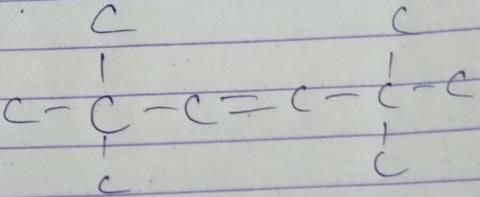QUESTION: 18

A hydrocarbon with molecular formula C10H18, upon catalytic hydrogenation gives C10H20 (X). X on free radical chlorination gives two monochloro derivatives with their molecular formula C10H19CI that are constitutional isomers.

Q. Which of the following reactions can synthesise X ?

Solution: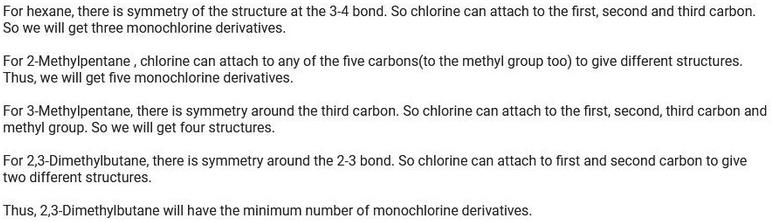*Answer can only contain numeric values
QUESTION: 19

Direction (Q. Nos, 19 - 22) This section contains 4 questions. When worked out will result in an integer from 0 to 9 (both inclusive).

Q. How many different isomers of alkenes {including stereoisomers) exist that all upon catalytic hydrogenation adds one mole of H2 to give the same 2, 2, 3,5-tetramethyl hexane?

Solution:
*Answer can only contain numeric values
QUESTION: 20

How many different monochlorination products would be obtained on free radical chlorination of methyl cyclobutane?

Solution:

After free radical halogenation of methyl cyclobutane, we have its 8different isomers. They are as follow:-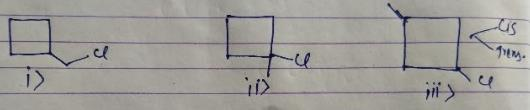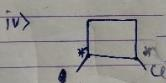From i) and ii), we get only positional isomers. From iii) we will have 2 isomers, cis and Trans. They won't show a chiral centre.
In iv) we have 2 chiral centres which will give us 4 isomers.So, in total there would be 4+2+1+1 = 8 isomers.

*Answer can only contain numeric values
QUESTION: 21

Theoretically, how many different monocarboxylic acids on heating with soda lime gives, 3-methyl pentane?

Solution:
*Answer can only contain numeric values
QUESTION: 22

From following list, how many of them upon catalytic hydrogenation would produce more heat than that produced in catalytic hydrogenation of frans-2-butene?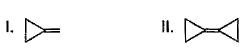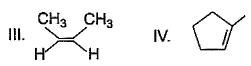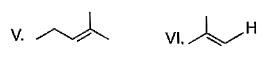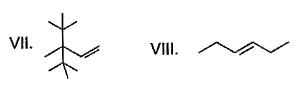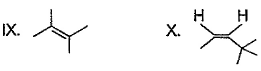Solution:

Correct answer is 4 because heat of hydrogenation is directly proportional to number of carbon atoms present in it

• Test